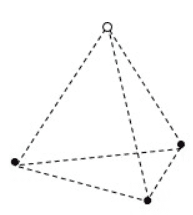# Find the Potential energy of a system of charges

Jaccobtw
Homework Statement:
3 electrons and a proton are arranged in a regular tetrahedron with a side length of 10nm. What is the total electrostatic potential energy of this configuration? Assume the energy is zero when the electrons are infinitely far from each other. Give your answer in J
Relevant Equations:
$$k \frac{q_1 q_2}{r}$$There are six pairs. three turn out to be negative and three turn out to be positive (3q^2 - 3q^2) which nets zero when you add them together with the equation. But zero was the incorrect answer. Did I do something wrong? Thank you

Homework Helper
Gold Member
2022 Award
But zero was the incorrect answer. Did I do something wrong?
You must have done. How did you try to calculate the PE?

Jaccobtw
You must have done. How did you try to calculate the PE?
$$\frac{k}{r}(3q^2 - 3q^2)$$ which is zero.

•PeroK
Homework Helper
Gold Member
2022 Award
$$\frac{k}{r}(3q^2 - 3q^2)$$ which is zero.
What is that?!

Jaccobtw
What is that?!
What's wrong? That's how you calculate the potential energy of a system of charges. You have 6 pairs. 3 of them are q^2 and 3 of them are -(q^2). You multiply them by k and divide by r and add them together to get an answer.

•PeroK
Homework Helper
Gold Member
I can't find anything incorrect with your calculation. How do you know that "zero" is incorrect?

•Jaccobtw
Homework Helper
Gold Member
2022 Award
What's wrong? That's how you calculate the potential energy of a system of charges. You have 6 pairs. 3 of them are q^2 and 3 of them are -(q^2). You multiply them by k and divide by r and add them together to get an answer.
Yes, sorry, I failed to see the remarkable symmetry with the 3:1 ratio.

•Jaccobtw
Jaccobtw
Yes, sorry, I failed to see the remarkable symmetry with the 3:1 ratio.
I didn't know symmetry was required.

Jaccobtw
I can't find anything incorrect with your calculation. How do you know that "zero" is incorrect?
The homework says it was wrong, but oddly enough the homework has been wrong many times this year